International
Tables for
Crystallography
Volume B
Reciprocal space
Edited by U. Shmueli

International Tables for Crystallography (2010). Vol. B, ch. 2.5, pp. 372-375   | 1 | 2 |

## Section 2.5.6.6. Direct Fourier inversion

B. K. Vainshteinc and P. A. Penczekg

#### 2.5.6.6. Direct Fourier inversion

| top | pdf |

Direct Fourier methods are based on the central section theorem (2.5.6.8). A set of the 2D Fourier transforms of projections yields an approximation to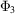on a nonuniform 3D grid, and a subsequent numerical 3D inverse Fourier transform gives an approximation to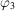. If the 3D inverse Fourier transform could be realized by means of the 3D inverse fast Fourier transform (FFT), one would have a very fast reconstruction algorithm. Unfortunately, the preprocessing step yieldson a nonuniform grid. In effect, the 3D inverse FFT is not applicable and an additional step of recovering samples ofon a uniform grid from the available samples on a nonuniform grid is necessary.

One possibility is to resample the nonuniformly sampled version ofonto a 3D Cartesian grid by some form of interpolation. For example, Grigorieff used a modified trilinear interpolation scheme in the FREALIGN package (Grigorieff, 1998). Simple interpolation methods have been found to give inaccurate results, although more sophisticated interpolation schemes can go a long way to improve the accuracy (Lanzavecchia et al., 1993). Unfortunately, the recommended window size makes them impractical for most applications.

The most accurate Fourier reconstruction methods are those that employ nonuniform Fourier transforms, particularly the 3D gridding method (O'Sullivan, 1985; Schomberg & Timmer, 1995). The gridding-based direct Fourier reconstruction algorithm (GDFR) (Penczek et al., 2004) was developed specifically for single-particle reconstruction. It comprises three steps:

 (1) The first step, known as gridding', involves calculating for the Fourier transform of each projection the convolution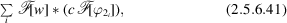where c are gridding weights' designed to compensate for the nonuniform distribution of the grid points and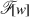is an appropriately chosen convolution kernel. After processing all projections, this step yields samples of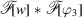on a Cartesian grid. (2) 3D inverse FFT is used to compute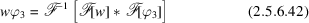on a Cartesian grid. (3) The weights are removed using division: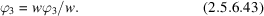The method involves a number of parameters. First, we need to decide the oversampling factor for the padding with zeros of input projections before FFTs are computed. In GDFR, the oversampling is set to two, although smaller factors can also yield good results. Second, we need a window functionwhose support in Fourier space is small'. In order to assure good computational efficiency of the convolution step (2.5.6.41), in GDFR this support was set to six Fourier voxels. In addition, in order to prevent division by zeros in (2.5.6.43), the weighting function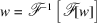must be positive within the support of the reconstructed object. A recommendable window function is the separable, bell-shaped Kaiser–Bessel window (O'Sullivan, 1985; Jackson et al., 1991; Schomberg & Timmer, 1995) (Fig. 2.5.6.6a):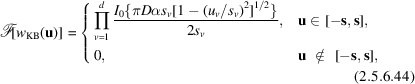where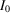is the zero-order modified Bessel function and α = 1.25 is a parameter. The weighting function associated with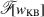is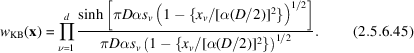Finally, the gridding weights c are chosen such that in discrete implementation of (2.5.6.41)and (2.5.6.42)we obtain a Riemann sum approximating the respective integral (Schomberg, 2002). The Voronoi diagram (Okabe et al., 2000) of the sampling points provides a good partitioning of sampling space, so the gridding weights c are computed by constructing a Voronoi diagram of the grid points and by choosing the weights as the volumes of the Voronoi cells (Fig. 2.5.6.7a). In cryo-EM, the number of projections, thus the number of sampling points in Fourier space, is extremely large. Thus, although the calculation of the gridding weights via the 3D Voronoi diagram of the nonuniformly spaced grid points for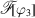would lead to an accurate direct Fourier method, the method would be very slow and would require excessive computer memory. To circumvent this problem, in GDFR (Penczek et al., 2004) the 2D reverse gridding method is used to compute the Fourier transform of each projection on a 2D polar grid. In this way,is obtained on a 3D spherical grid, where the grid points are located both on centred spheres and on straight lines through the origin. Accordingly, it becomes possible to partition the sampled region into suitable sampling cells via the computation of a 2D Voronoi diagram on a unit sphere, rather than a 3D Voronoi diagram in Euclidean space (Fig. 2.5.6.7b). This significantly reduces the memory requirements and improves the computational efficiency of the method, particularly when a fast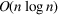algorithm for computing the Voronoi diagram on the unit sphere is employed (Renka, 1997).Figure 2.5.6.6 | top | pdf |Kaiser–Bessel window function used in the gridding reconstruction algorithm GDFR. (a) In Fourier space, the window function is effectively zero outside the support of six Fourier pixels. (b) In real space, the zeros of the window function are beyond the radius of the reconstructed object.Figure 2.5.6.7 | top | pdf |Partitions of the sampling Fourier space using Voronoi diagrams in direct inversion algorithms. (a) Central section of a 3D Fourier volume with sampling points originating from 2D Fourier transforms of projections. Although 2D projections are sampled on a uniform (Cartesian) grid, the arbitrary rotations of projections in 3D space yields a nonuniform distribution of points in three dimensions. In effect, the 3D reconstruction by direct inversion using 3D FFT is not possible. (b) Voronoi diagram on a sphere in the GDFR algorithm. Using the reverse gridding method, the 2D Fourier transforms of projections are resampled onto 1D central lines using a constant angular step. In 3D Fourier space, they are located on central sections and their angular directions are evenly distributed on grand circles. However, since central sections have nonuniform distributions, the distribution of angular directions (sampling points on the unitary sphere) is also nonuniform and effectively random.

The gridding weights in GDFR correspond to the weighting functions in filtered backprojection algorithms. Moreover, setting the gridding weights to be equal to angular regions on the unit sphere directly corresponds to the equating of weighting function (2.5.6.29)in 2D filtered backprojection to the length of an arc on a unitary circle. Thus, both methods yield nearly optimum projection density functions.

The reversed gridding method is obtained by reversing the sequence of steps (1)–(3)of the gridding method:

 (1) the input image is divided by the weighting function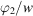; (2) the image is padded with zeros and 2D FFT is used to compute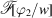; (3) gridding is used to compute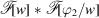on an arbitrary nonuniform grid.

Note the reverse gridding does not require explicit gridding weights, as in the third step they are constant (Penczek et al., 2004).

In GDFR, the third step of the reversed gridding results in a set of 1D Fourier central lines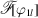calculated using a constant angular step. Clearly, upon inverse Fourier transform they amount to a Radon transform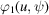of the projection and, upon repeating the process for all available projections, they yield a Radon transform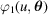of a 3D object(albeit non­uniformly sampled with respect to Eulerian angles). Thus GDFR, in addition to being a method of inverting a 3D ray transform, is also a highly accurate method of inverting a 3D Radon transform. GDFR is implemented in the SPIDER package (Frank et al., 1996).

The results of a comparison of selected reconstruction algorithms are shown in Fig. 2.5.6.8. The tests were performed using simulated data with the projections of a phantom 3D structure calculated using the inverse gridding method (Penczek et al., 2004). The GDFR yields a virtually perfect reconstruction that agrees with the phantom over the whole frequency range (with the exception of the highest frequencies, but these cannot be reproduced due to geometrical limitations). GD3D is also a gridding-based reconstruction algorithm, in which the gridding weights are calculated directly from contributions of the weighting function to the 3D Fourier pixels and are applied to the voxels in the 3D Fourier volume (Jackson et al., 1991). This yields an approximation to the local density' of contributing projections. The simplified gridding weights in GD3D result in deterioration of the reconstruction in the low- and intermediate-frequency ranges. The general weighted backprojection with exponent-based weighting function (WBP1) performs quite well, although reproduction in the low-frequency range is inferior. Similar artifacts are present in the reconstruction using the exact filter weighted backprojection (WBP2), which, in addition, performs disappointingly at higher frequencies. This relatively poor performance is attributed to the nonoptimal weighting schemes used in both methods. It is also interesting to note that the backprojection step is identical in both algorithms; they only differ by the weighting function. The significant difference in their performance attests to the importance of good weighting schemes for high-quality 3D reconstructions from nonuniformly distributed projections. SIRT yields a reconstruction that in the low- and intermediate-frequency ranges matches in quality the reconstruction obtained with GDFR. However, there is a significant loss of quality at high frequencies. This is due to the inferior linear interpolation scheme used in SIRT (this impediment is shared with WBP1 and WBP2). [The dip of the SIRT fidelity curve at a spatial frequency of 0.42 in Fig. 2.5.6.8(a) is due to an inconsistency between the interpolation methods used internally in SIRT to generate intermediate projections of the object to be reconstructed and the Fourier-space-based method used to generate the test data.] In terms of computational efficiency, gridding-based algorithms outperform weighted backprojection algorithms by a small factor while SIRT is approximately ten times slower (depending on the number of iterations used).Figure 2.5.6.8 | top | pdf |(a) Plot of correlation coefficients (CCs) calculated between Fourier transforms of the reconstructed structure and the original phantom as a function of the magnitude of the spatial frequency using five reconstruction algorithms. GDFR: gridding direct Fourier reconstruction with Voronoi weights; GD3D: gridding reconstruction with simplified gridding weights; WBP1: general weighted backprojection with exponent-based weighting function; WBP2: exact filter weighted backprojection; SIRT: simultaneous iterative reconstruction algorithm. Noise-free projection data were computed in Fourier space using the reverse gridding method. (b) Rescaled version of the low-frequency range of (a). Note the different scales in (a) and (b). The horizontal axis is scaled in absolute frequency units with 0.5 equal to the Nyquist frequency.

### References

Frank, J., Radermacher, M., Penczek, P., Zhu, J., Li, Y., Ladjadj, M. & Leith, A. (1996). SPIDER and WEB: processing and visualization of images in 3D electron microscopy and related fields. J. Struct. Biol. 116, 190–199.
Grigorieff, N. (1998). Three-dimensional structure of bovine NADH: ubiquinone oxidoreductase (complex I) at 22 Å in ice. J. Mol. Biol. 277, 1033–1046.
Jackson, J. I., Meyer, C. H., Nishimura, D. G. & Macovski, A. (1991). Selection of a convolution function for Fourier inversion using gridding. IEEE Trans. Med. Imaging, 10, 473–478.
Lanzavecchia, S., Bellon, P. L. & Scatturin, V. (1993). SPARK, a kernel of software programs for spatial reconstruction in electron microscopy. J. Microsc. 171, 255–266.
O'Sullivan, J. D. (1985). A fast sinc function gridding algorithm for Fourier inversion in computer tomography. IEEE Trans. Med. Imaging, 4, 200–207.
Okabe, A., Boots, B., Sugihara, K. & Chiu, S. N. (2000). Spatial Tessellations: Concepts and Applications of Voronoi Diagrams. New York: John Wiley & Sons.
Penczek, P. A., Renka, R. & Schomberg, H. (2004). Gridding-based direct Fourier inversion of the three-dimensional ray transform. J. Opt. Soc. Am. A, 21, 499–509.
Renka, R. J. (1997). Algorithm 772. STRIPACK: Delaunay triangulation and Voronoi diagram on the surface of a sphere. ACM Trans. Math. Software, 23, 416–434.
Schomberg, H. (2002). Notes on direct and gridding-based Fourier inversion methods. In Proceedings of the IEEE International Symposium on Biomedical Imaging, Washington, DC, edited by M. Unser & Z. P. Liang, pp. 645–648.
Schomberg, H. & Timmer, J. (1995). The gridding method for image reconstruction by Fourier transformation. IEEE Trans. Med. Imaging, 14, 596–607.